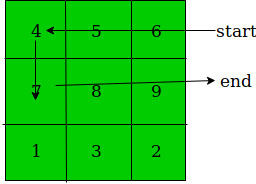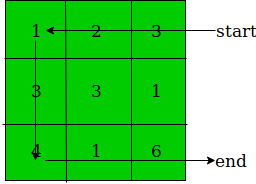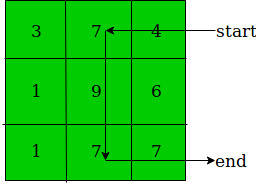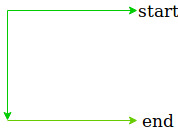# Find maximum path sum in a 2D matrix when exactly two left moves are allowed

Given a 2D matrix arr[][] of dimensions N * M where N is number of rows and M is number of columns.The task is to find maximum path sum in this matrix satisfying some condition which are as follows :

1. We can only start with arr[i][M] where 0 <= i <= N.
2. We end the path on the same side, such that we can take exactly 2 left turns.
3. Example:Examples:

```Input : N = 3, M = 3
arr[][] = {{1, 2, 3},
{3, 3, 1},
{4, 1, 6}}
Output : 20
Explanation :If we follow this path then we get the sum 20.

Input : N = 3, M = 3
arr[][] = {{3, 7, 4},
{1, 9, 6},
{1, 7, 7}}
Output : 34
Explanation :If we follow this path then we get the sum 34.
```

## Recommended: Please try your approach on {IDE} first, before moving on to the solution.

The idea is to use dynamic programming and select an optimal structure in the matrix i.e.
C shaped structure as shown in the below image.Steps are as follows :

1. First we calculate suffix sum in each row and store it in another 2D matrix call it b[][]so that at every valid index we get the sum of the entire row starting from that index.

b[i][j] = arr[i][j] + b[i][j + 1]

2. Now we check each consecutive two rows and find the sum of their corresponding columns and simultaneously updating the maximum sum variable. Till now we have found both horizontal lines from that above structure.

sum = max(sum, b[i][j] + b[i – 1][j])

We need to find that vertical line connecting these horizontal lines i.e. column.

3. After traversing each row, for each valid index we have two choices either we link this index to corresponding index of upper row i.e. add in previous column or start a new column.
Whichever value is maximum we retain that value and we update the value at this index.

b[i][j] = max(b[i][j], b[i – 1][j] + arr[i][j])

Below is the implementation of the above approach:

## C++

 `// C++ program to find maximum path sum ` `// in a 2D matrix when exactly two ` `// left moves are allowed ` `#include ` `#define N 3 ` `#define M 3 ` `using` `namespace` `std; ` ` `  `// Function to return the maximum path sum ` `int` `findMaxSum(``int` `arr[][M]) ` `{ ` `    ``int` `sum = 0; ` `    ``int` `b[N][M]; ` `     `  `    ``// Copy last column i.e. starting and  ` `    ``// ending columns in another array ` `    ``for` `(``int` `i = 0; i < N; i++) { ` `        ``b[i][M - 1] = arr[i][M - 1]; ` `    ``} ` `     `  `    ``// Calculate suffix sum in each row ` `    ``for` `(``int` `i = 0; i < N; i++) { ` `        ``for` `(``int` `j = M - 2; j >= 0; j--) { ` `            ``b[i][j] = arr[i][j] + b[i][j + 1]; ` `        ``} ` `    ``} ` `     `  `    ``// Select the path we are going to follow ` `    ``for` `(``int` `i = 1; i < N; i++) { ` `        ``for` `(``int` `j = 0; j < M; j++) { ` `            ``sum = max(sum, b[i][j] + b[i - 1][j]); ` `             `  `            ``b[i][j] = max(b[i][j], b[i - 1][j] + arr[i][j]); ` `        ``} ` `    ``} ` `     `  `    ``return` `sum; ` `} ` ` `  `// Driver Code ` `int` `main() ` `{ ` `    ``int` `arr[N][M] = {{ 3, 7, 4 },  ` `                     ``{ 1, 9, 6 },  ` `                     ``{ 1, 7, 7 }}; ` `                      `  `    ``cout << findMaxSum(arr) << endl; ` ` `  `    ``return` `0; ` `} `

## Java

 `// Java program to find maximum path sum ` `// in a 2D matrix when exactly two ` `// left moves are allowed ` `import` `java.io.*; ` ` `  `class` `GFG  ` `{ ` `     `  `static` `int` `N = ``3``; ` `static` `int` `M = ``3``; ` ` `  `// Function to return the maximum path sum ` `static` `int` `findMaxSum(``int` `arr[][]) ` `{ ` `    ``int` `sum = ``0``; ` `    ``int` `[][]b = ``new` `int` `[N][M]; ` `     `  `    ``// Copy last column i.e. starting and  ` `    ``// ending columns in another array ` `    ``for` `(``int` `i = ``0``; i < N; i++)  ` `    ``{ ` `        ``b[i][M - ``1``] = arr[i][M - ``1``]; ` `    ``} ` `     `  `    ``// Calculate suffix sum in each row ` `    ``for` `(``int` `i = ``0``; i < N; i++)  ` `    ``{ ` `        ``for` `(``int` `j = M - ``2``; j >= ``0``; j--)  ` `        ``{ ` `            ``b[i][j] = arr[i][j] + b[i][j + ``1``]; ` `        ``} ` `    ``} ` `     `  `    ``// Select the path we are going to follow ` `    ``for` `(``int` `i = ``1``; i < N; i++)  ` `    ``{ ` `        ``for` `(``int` `j = ``0``; j < M; j++)  ` `        ``{ ` `            ``sum = Math.max(sum, b[i][j] + b[i - ``1``][j]); ` `             `  `            ``b[i][j] = Math.max(b[i][j], b[i - ``1``][j] + arr[i][j]); ` `        ``} ` `    ``} ` `     `  `    ``return` `sum; ` `} ` ` `  `// Driver Code ` `public` `static` `void` `main (String[] args)  ` `{ ` ` `  `    ``int` `arr[][] = {{ ``3``, ``7``, ``4` `},  ` `                    ``{ ``1``, ``9``, ``6` `},  ` `                    ``{ ``1``, ``7``, ``7` `}}; ` `                 `  `    ``System.out.println (findMaxSum(arr)); ` `} ` `} ` ` `  `// This code is contributed by ajit. `

## Python3

 `# Python3 program to find maximum path sum  ` `# in a 2D matrix when exactly two  ` `# left moves are allowed  ` `import` `numpy as np ` `N ``=` `3` `M ``=` `3` ` `  `# Function to return the maximum path sum  ` `def` `findMaxSum(arr) :  ` ` `  `    ``sum` `=` `0``;  ` `    ``b ``=` `np.zeros((N, M));  ` `     `  `    ``# Copy last column i.e. starting and  ` `    ``# ending columns in another array  ` `    ``for` `i ``in` `range``(N) :  ` `        ``b[i][M ``-` `1``] ``=` `arr[i][M ``-` `1``];  ` `     `  `    ``# Calculate suffix sum in each row  ` `    ``for` `i ``in` `range``(N) : ` `        ``for` `j ``in` `range``(M ``-` `2``, ``-``1``, ``-``1``) :  ` `            ``b[i][j] ``=` `arr[i][j] ``+` `b[i][j ``+` `1``];  ` `     `  `    ``# Select the path we are going to follow  ` `    ``for` `i ``in` `range``(``1``, N) : ` `        ``for` `j ``in` `range``(M) : ` `            ``sum` `=` `max``(``sum``, b[i][j] ``+` `b[i ``-` `1``][j]);  ` `             `  `            ``b[i][j] ``=` `max``(b[i][j],  ` `                          ``b[i ``-` `1``][j] ``+` `arr[i][j]); ` `             `  `    ``return` `sum``;  ` ` `  `# Driver Code  ` `if` `__name__ ``=``=` `"__main__"` `:  ` ` `  `    ``arr ``=` `[[ ``3``, ``7``, ``4` `],  ` `           ``[ ``1``, ``9``, ``6` `],  ` `           ``[ ``1``, ``7``, ``7` `]];  ` `                     `  `    ``print``(findMaxSum(arr));  ` ` `  `# This code is contributed by AnkitRai01 `

## C#

 `// C# program to find maximum path sum ` `// in a 2D matrix when exactly two ` `// left moves are allowed ` `using` `System; ` `     `  `class` `GFG  ` `{ ` `     `  `static` `int` `N = 3; ` `static` `int` `M = 3; ` ` `  `// Function to return the maximum path sum ` `static` `int` `findMaxSum(``int` `[,]arr) ` `{ ` `    ``int` `sum = 0; ` `    ``int` `[,]b = ``new` `int` `[N, M]; ` `     `  `    ``// Copy last column i.e. starting and  ` `    ``// ending columns in another array ` `    ``for` `(``int` `i = 0; i < N; i++)  ` `    ``{ ` `        ``b[i, M - 1] = arr[i, M - 1]; ` `    ``} ` `     `  `    ``// Calculate suffix sum in each row ` `    ``for` `(``int` `i = 0; i < N; i++)  ` `    ``{ ` `        ``for` `(``int` `j = M - 2; j >= 0; j--)  ` `        ``{ ` `            ``b[i, j] = arr[i, j] + b[i, j + 1]; ` `        ``} ` `    ``} ` `     `  `    ``// Select the path we are going to follow ` `    ``for` `(``int` `i = 1; i < N; i++)  ` `    ``{ ` `        ``for` `(``int` `j = 0; j < M; j++)  ` `        ``{ ` `            ``sum = Math.Max(sum, b[i, j] + b[i - 1, j]); ` `             `  `            ``b[i, j] = Math.Max(b[i, j], b[i - 1, j] + arr[i, j]); ` `        ``} ` `    ``} ` `     `  `    ``return` `sum; ` `} ` ` `  `// Driver Code ` `public` `static` `void` `Main ()  ` `{ ` ` `  `    ``int` `[,]arr = {{ 3, 7, 4 },  ` `                    ``{ 1, 9, 6 },  ` `                    ``{ 1, 7, 7 }}; ` `                 `  `    ``Console.WriteLine(findMaxSum(arr)); ` `} ` `} ` ` `  `/* This code contributed by PrinciRaj1992 */`

Output:

```34
```

Time Complexity :My Personal Notes arrow_drop_upCheck out this Author's contributed articles.

If you like GeeksforGeeks and would like to contribute, you can also write an article using contribute.geeksforgeeks.org or mail your article to contribute@geeksforgeeks.org. See your article appearing on the GeeksforGeeks main page and help other Geeks.

Please Improve this article if you find anything incorrect by clicking on the "Improve Article" button below.